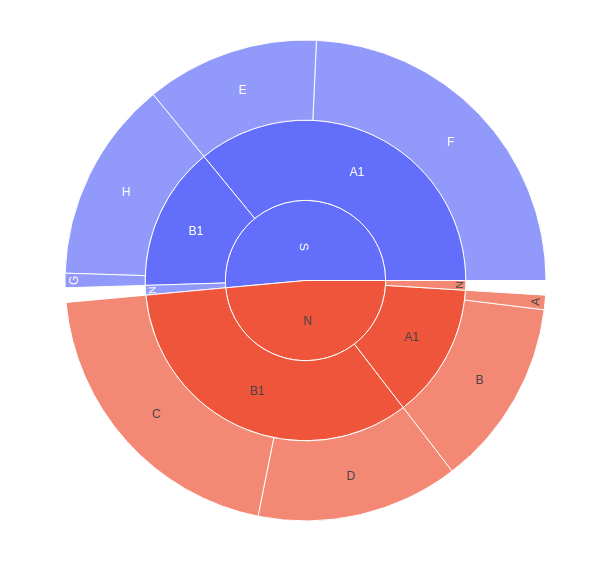Open In App

# Sunburst Plot using Plotly in Python

Plotly is a Python library that is used to design graphs, especially interactive graphs. It can plot various graphs and charts like histogram, barplot, boxplot, spreadplot, and many more. It is mainly used in data analysis as well as financial analysis. plotly is an interactive visualization library.

## Sunburst Plot in Plotly

Sunburst plot visualizes stratified data gradually from roots to leaves. The root starts from the center and squirt are added to the outer rings. Each level of the hierarchy is represented by one ring or circle with the innermost circle, further rings are divided into slices that represent data points and the size of the slice represents data values.

Syntax: plotly.express.sunburst(data_frame=None, names=None, values=None, parents=None, path=None, ids=None, color=None, color_continuous_scale=None, range_color=None, color_continuous_midpoint=None, color_discrete_sequence=None, color_discrete_map={}, hover_name=None, hover_data=None, custom_data=None, labels={}, title=None, template=None, width=None, height=None, branchvalues=None, maxdepth=None)

Parameters:

data_frame: This argument needs to be passed for column names (and not keyword names) to be used.

names: Either a name of a column in data_frame, or a pandas Series or array_like object. Values from this column or array_like are used as labels for sectors.

values:  Either a name of a column in data_frame or a pandas Series or array_like object. Values from this column or array_like are used to set values associated to sectors.

parents: Either a name of a column in data_frame, or a pandas Series or array_like object. Values from this column or array_like are used as parents in sunburst and treemap charts.

path: Either names of columns in data_frame, or pandas Series, or array_like objects List of columns names or columns of a rectangular dataframe defining the hierarchy of sectors, from root to leaves.

ids: Either a name of a column in data_frame, or a pandas Series or array_like object. Values from this column or array_like are used to set ids of sectors

Example:

## Python3

 `import` `plotly.express as px`` ` `df ``=` `px.data.iris()`` ` `fig ``=` `px.sunburst(df, path``=``[``'sepal_length'``, ``                            ``'sepal_width'``,``                            ``'petal_length'``], ``                  ``values``=``'petal_width'``)``fig.show()`

Output: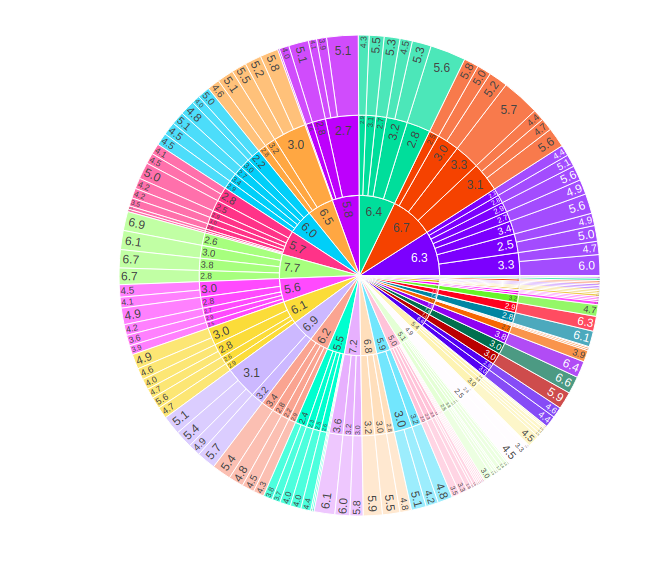## Plotting hierarchical data

The rectangular dataframe represents the hierarchical data where different columns correspond to different levels of hierarchy. To plot such columns path parameter is used. Path parameter takes either name of columns in data_frame, or pandas Series, or array_like objects, list of columns names or columns of a rectangular dataframe defining the hierarchy of sectors, from root to leaves.

Note: When ids or parents are passed along with path an error is raised.

Example:

## Python3

 `import` `plotly.express as px`` ` `df ``=` `px.data.tips()`` ` `fig ``=` `px.sunburst(df, path``=``[``'day'``, ``'sex'``], ``                  ``values``=``'total_bill'``)``fig.show()`

Output: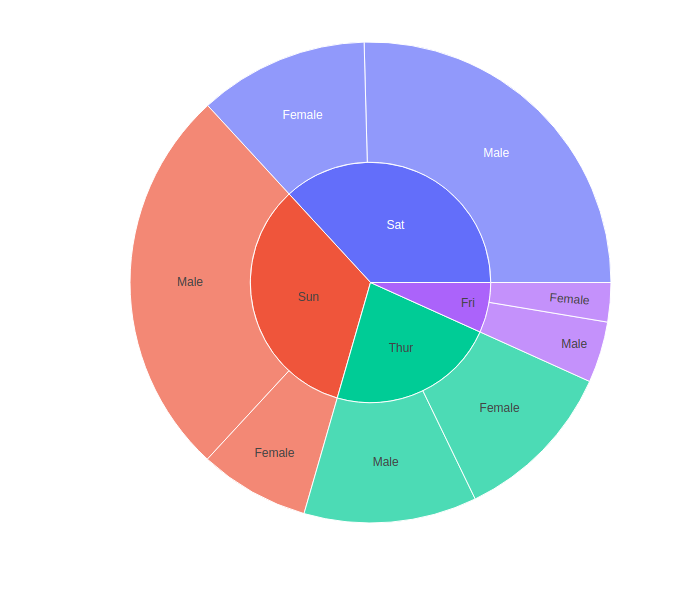### Plotting hierarchical data with the continuous color argument

If the color argument is passed, the color of the node is calculated as the average color values of its children by their values.

Example:

## Python3

 `import` `plotly.express as px`` ` `df ``=` `px.data.tips()`` ` `fig ``=` `px.sunburst(df, path``=``[``'day'``, ``'sex'``], ``                  ``values``=``'total_bill'``, color``=``'total_bill'``)``fig.show()`

Output: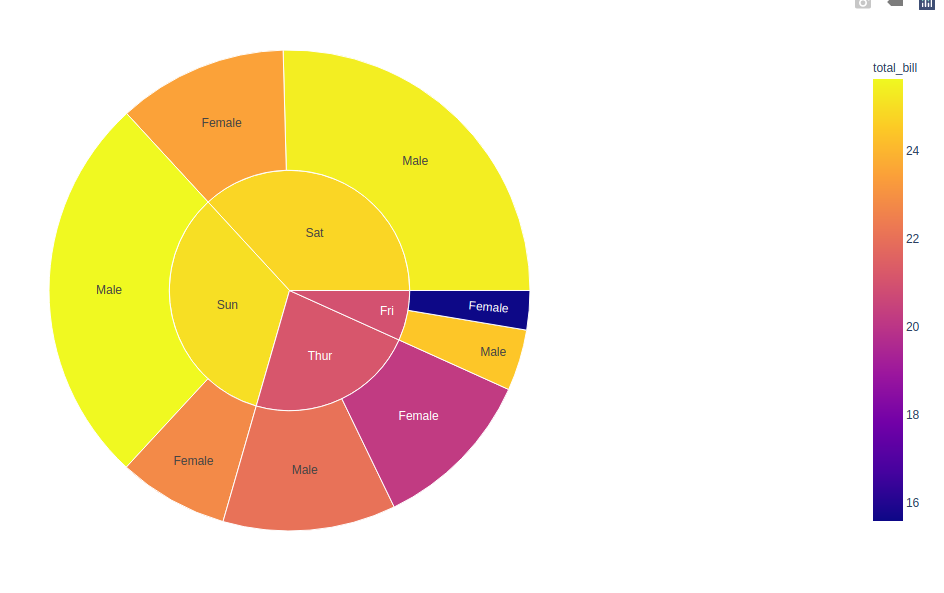### Plotting hierarchical data with the discrete color argument

When non-numerical data is passed to the color argument, then discrete data is used. If a color column of a sector has the same value for all of its children, then the corresponding color is used otherwise the same first color of discrete color will be used.

Example:

## Python3

 `import` `plotly.express as px`` ` `df ``=` `px.data.tips()`` ` `fig ``=` `px.sunburst(df, path``=``[``'day'``, ``'sex'``], ``                  ``values``=``'total_bill'``, color``=``'time'``)``fig.show()`

Output: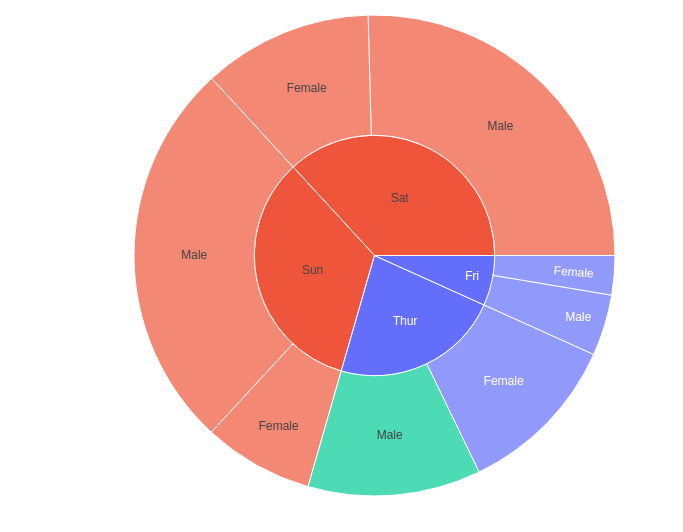### Plotting hierarchical data with missing values

If the data-set is not fully rectangular in shape, then missing values should be mentioned as none. None entries of the parents must be a leaf, otherwise, valueError will be raised.

Example:

## Python3

 `import` `plotly.express as px``import` `pandas as pd`` ` ` ` `A ``=` `[``"A"``, ``"B"``, ``"C"``, ``"D"``, ``None``, ``"E"``,``           ``"F"``, ``"G"``, ``"H"``, ``None``]`` ` `B ``=` `[``"A1"``, ``"A1"``, ``"B1"``, ``"B1"``, ``"N"``,``           ``"A1"``, ``"A1"``, ``"B1"``, ``"B1"``, ``"N"``]``C ``=` `[``"N"``, ``"N"``, ``"N"``, ``"N"``, ``"N"``,``           ``"S"``, ``"S"``, ``"S"``, ``"S"``, ``"S"``]``D ``=` `[``1``, ``13``, ``21``, ``14``, ``1``, ``12``, ``25``, ``1``, ``14``, ``1``]`` ` `df ``=` `pd.DataFrame(``    ``dict``(A``=``A, B``=``B, C``=``C, D``=``D)``)`` ` `fig ``=` `px.sunburst(df, path``=``[``'C'``, ``'B'``, ``'A'``], values``=``'D'``)``fig.show()`

Output: